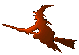Hex  1.0 Hydrogen-electron collision solver
GaussLegendre Class Reference

Gauss-Legendre quadrature. More...

#include <gauss.h>

## Public Member Functions

GaussLegendre (Bspline const &bspline)

int gauss_nodes_and_weights (int points, const double *&vx, const double *&vw) const
Retrieve Gauss-Legendre data. More...

cArray p_points (int points, Complex x1, Complex x2) const
Get Gauss-Legendre quadrature points in interval. More...

cArray p_weights (int points, Complex x1, Complex x2) const
Get Gauss-Legendre quadrature weights in interval. More...

template<class Functor , class... Data>
Complex quad (Functor f, int points, int iknot, Complex x1, Complex x2, Data...data) const
Gauss-Legendre integrator. More...

template<class ClassPtr , class Functor , class... Data>
Complex quadMFP (ClassPtr ptr, Functor f, int points, int iknot, Complex x1, Complex x2, Data...data) const
Gauss-Legendre integrator. More...

## Detailed Description

This class's purpose is aid in fixed-order Gauss-Legendre quadrature. It is constructed above a B-spline environment, which is passed to the constructor. The functions "p_points" and "p_weights" return evaluation nodes and weights, respectively, and the functions "quad" do the fixed-order quadrature itself. Every call to p_points or p_weights resuts in call to gauss_nodes_and_weights, which uses GSL to get the requested data. The computed nodes and weights are stored in a cache table to allow faster subsequent computations.

## Constructor & Destructor Documentation

 GaussLegendre::GaussLegendre ( Bspline const & bspline )
inline

## Member Function Documentation

 int GaussLegendre::gauss_nodes_and_weights ( int points, const double *& vx, const double *& vw ) const

Precompute Gauss-Legendre quadrature data (if not already done is some previous call) and return pointers to the chache table.

Parameters
 points Gauss-Legendre points half-count. If too low/high, the return value will contain the (used) nearest implemented value. vx On return, the Gauss-Legendre nodes (nonnegative half of them). vw On return, the corresponding Gauss-Legendre weights.
 cArray GaussLegendre::p_points ( int points, Complex x1, Complex x2 ) const

Map Gauss-Legendre points provided by gauss_nodes_and_weights to a complex interval $$(x_1,x_2)$$.

Parameters
 points Number of Gauss-Legendre points. x1 Left boundary of the interval. x2 Right boundary of the interval.
 cArray GaussLegendre::p_weights ( int points, Complex x1, Complex x2 ) const

Map Gauss-Legendre weights provided by gauss_nodes_and_weights to a complex interval $$(x_1,x_2)$$.

Parameters
 points Number of Gauss-Legendtre points. x1 Left boundary of the interval. x2 Right boundary of the interval.
template<class Functor , class... Data>
 Complex GaussLegendre::quad ( Functor f, int points, int iknot, Complex x1, Complex x2, Data... data ) const
inline

Integrate the given function.

Parameters
 f Function of type (void (*) (unsigned, complex*, complex*, data...)), where the first parameter is number of points to evaluate, the second parameter is pointer to an array of double complex values at which to evaluate, the third is pointer to an array into which the data will be written (responsibility for memory management is on callers side) and finally the fourth (and further) parameter is any other data to be suplied to the function. data Data to pass to the function. points Gauss-Legendre points count. iknot Knot index. x1 Left integration boundary. x2 Right integration boundary.

It must be

* t[iknot] <= x1 <= t[iknot+1]
* t[iknot] <= x2 <= t[iknot+1]
*
template<class ClassPtr , class Functor , class... Data>
 Complex GaussLegendre::quadMFP ( ClassPtr ptr, Functor f, int points, int iknot, Complex x1, Complex x2, Data... data ) const
inline

This is a variant of the other quad function that can be used together with the pointer-to-member-function arguments. A typical usage woud be

* Class A { ... };
* A a;
* result = quad (&a, &a::integrand, ...);
*

The documentation for this class was generated from the following files: